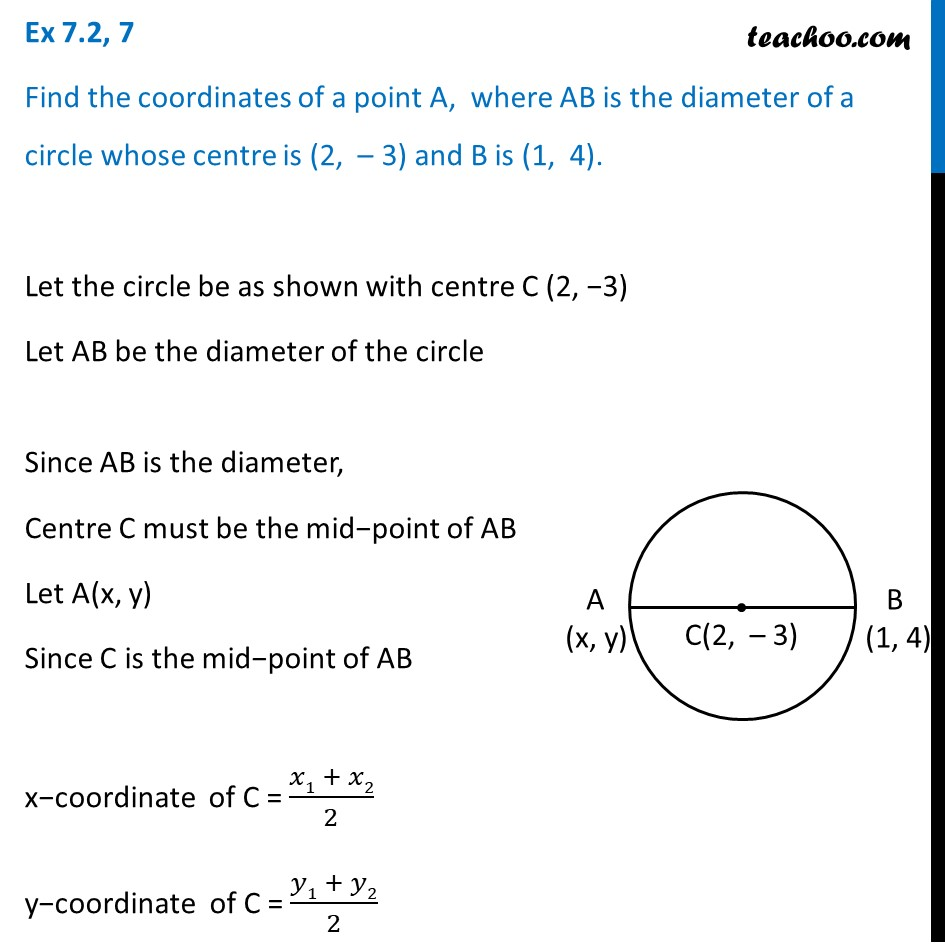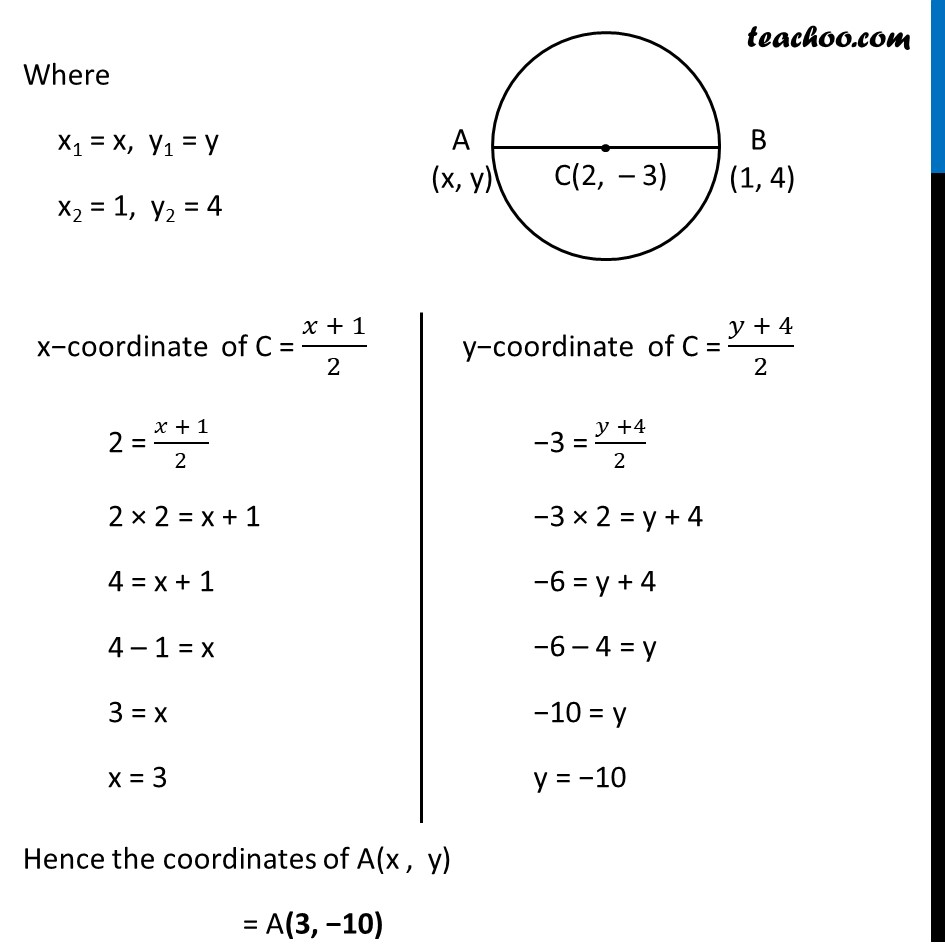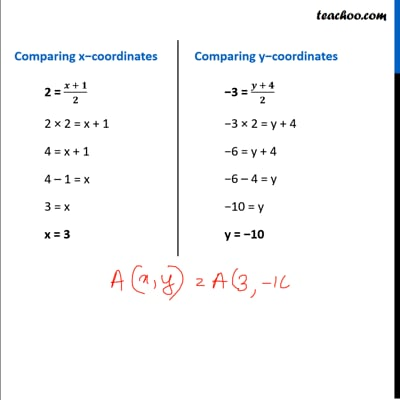Ex 7.2

Chapter 7 Class 10 Coordinate Geometry
Serial order wiseThis video is only available for Teachoo black users

### Transcript

Ex 7.2, 7 Find the coordinates of a point A, where AB is the diameter of a circle whose centre is (2, – 3) and B is (1, 4). Let the circle be as shown with centre C (2, −3) Let AB be the diameter of the circle Since AB is the diameter, Centre C must be the mid−point of AB Let A(x, y) Since C is the mid−point of AB x−coordinate of C = (𝑥1 + 𝑥2)/2 y−coordinate of C = (𝑦1 + 𝑦2)/2 Where x1 = x, y1 = y x2 = 1, y2 = 4 Hence the coordinates of A(x , y) = A(3, −10) x−coordinate of C = (𝑥 + 1)/2 2 = (𝑥 + 1)/2 2 × 2 = x + 1 4 = x + 1 4 – 1 = x 3 = x x = 3 y−coordinate of C = (𝑦 + 4)/2 −3 = (𝑦 +4)/2 −3 × 2 = y + 4 −6 = y + 4 −6 – 4 = y −10 = y y = −10